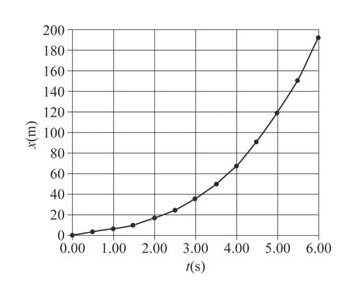# A race car moves such that its position fits the relationshipx=  (5.0 m/s)t +  (0.75 m/s3)t3where x is measured in meters and t in seconds. (a) Plot agraph of the car’s position versus time. (b) Determine theinstantaneous velocity of the car at t = 4.0 s, using time intervalsof 0.40 s, 0.20 s, and 0.10 s. (c) Compare the averagevelocity during the first 4.0 s with the results of part (b).

Question
1 views

A race car moves such that its position fits the relationship
x=  (5.0 m/s)t +  (0.75 m/s3)t3
where x is measured in meters and t in seconds. (a) Plot a
graph of the car’s position versus time. (b) Determine the
instantaneous velocity of the car at t = 4.0 s, using time intervals
of 0.40 s, 0.20 s, and 0.10 s. (c) Compare the average
velocity during the first 4.0 s with the results of part (b).

check_circle

Step 1

Given,

The position of the car, x = (5.0m/s)t+(0.75m/s3)t3

Step 2

(a)

The position versus time graph of the car is plotted below.help_outlineImage Transcriptionclose200 180 160 140 120 100 80 60 40 20 1.00 6.00 0.00 2.00 3.00 4.00 5.00 1(s) (u)x fullscreen
Step 3

(b)

Consider a time interval of 0.40s

At t=3.80s, x=60.2m

At t=4.20...

### Want to see the full answer?

See Solution

#### Want to see this answer and more?

Solutions are written by subject experts who are available 24/7. Questions are typically answered within 1 hour.*

See Solution
*Response times may vary by subject and question.
Tagged in

### Kinematics